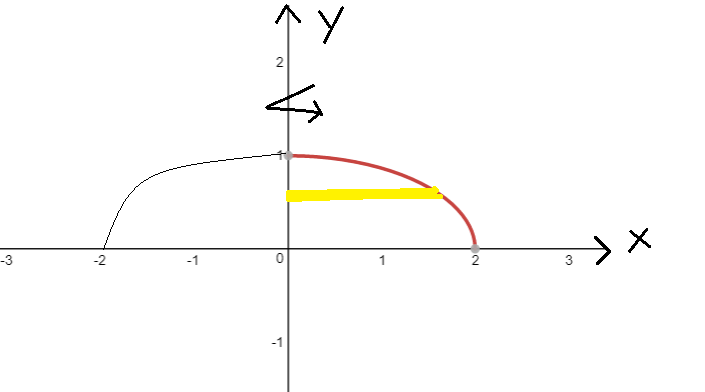# find the volume of the solid generated by revolving the region bounded by the given curves about the y-axis. Use the disks methodx^2 + 4y^2=4 (quadrant I)

Question
24 views

find the volume of the solid generated by revolving the region bounded by the given curves about the y-axis. Use the disks method

check_circle

star
star
star
star
star
1 Rating
Step 1

We have been given the portion of ellipse x^2+4y^2=4 in first quadrant. It is being rotated about y axis and we are supposed to find volume of the formed solid. Let us first understand how the shape would look like after graphing.Step 2

Using disk method, we can set up the integral for volume...

### Want to see the full answer?

See Solution

#### Want to see this answer and more?

Solutions are written by subject experts who are available 24/7. Questions are typically answered within 1 hour.*

See Solution
*Response times may vary by subject and question.
Tagged in

### Integration